Select Page

# Maths 12 Science Relations and Functions CBSE Answers for MCQ in English

Maths 12 Science Relations and Functions CBSE Answers for MCQ in English to enable students to get Answers in a narrative video format for the specific question.

Expert Teacher provides Maths 12 Science Relations and Functions CBSE Answers for MCQ through Video Answers in English language. This video solution will be useful for students to understand how to write an answer in exam in order to score more marks. This teacher uses a narrative style for a question from Relations and Functions not only to explain the proper method of answering question, but deriving right answer too.

Please find the question below and view the Answer in a narrative video format.

Question:

## Similar Questions from CBSE, 12th Science, Maths, Relations and Functions

Question 1 : Functions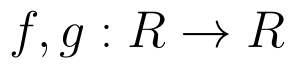are defined respectively, by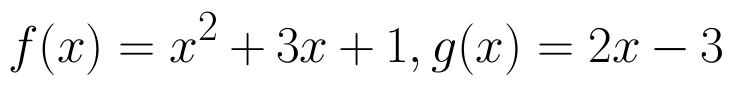, find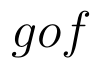(View Answer Video)

Question 2 : The identity element for the binary operation * defined by a * b =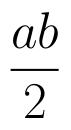, where a, b are the elements of a set of non-zero rational numbers, is, (View Answer Video)

Question 3 : A function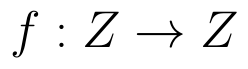defined as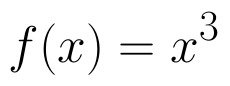is, (View Answer Video)

Question 4 : If f is the greatest integer function and g is the modulus function . Write the value of g o f(-1/3) – f o g ( -1/3 ). (View Answer Video)

Question 5 : A functiondefined as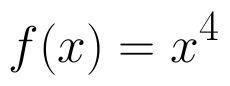is, (View Answer Video)

### Linear Programming

Question 1 : The objective function is maximum or minimum, which lies on the boundary of the feasible region. (View Answer Video)

### Differential Equations

Question 1 : Find the particular solution of the differential equation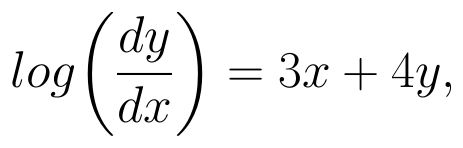given that y = 0, when x = 0. (View Answer Video)

Question 2 : Form the differential equation of equation y = a cos 2x + b sin 2x, where a and b are constant. (View Answer Video)

Question 3 : Write the degree of the differential equation :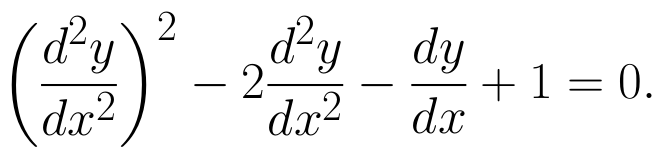(View Answer Video)

Question 4 : Form the differential equation of the family of circles touching the x-axis at origin .  (View Answer Video)

Question 5 : Write the differential equation obtained by eliminating the arbitrary constant C in the equation representing the family of curves xy = C cos x. (View Answer Video)

### Application of Integrals

Question 1 : Find the area of the region bounded by the parabola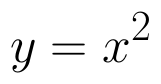and y = | x |. (View Answer Video)

Question 2 : Using the method of integration, find the area of the region bounded by the lines: 5x - 2y -10 = 0, x + y - 9 = 0 and 2x - 5y - 4 = 0. (View Answer Video)

Question 3 : Find the area of the given curves and given lines: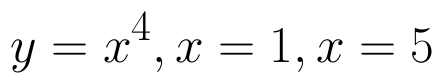and x-axis (View Answer Video)

Question 4 : Find the area of the region enclosed by the parabola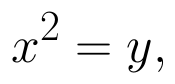the line y = x + 2. (View Answer Video)

Question 5 : Find the area bounded by the curve y = sin x between x = 0 and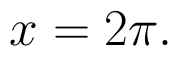(View Answer Video)